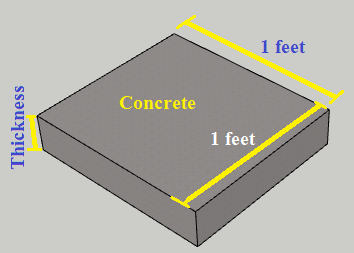# Concrete Calculator to Estimate the Concrete Materials

## Enter Dimension of Concrete to Construct## How to use this tool?

Concrete Calculator calculates the volume of cement, sand, and aggregate, the weight of steel rod according to percentage, Numbers of bags of cement required to construct cubical structural members like Beam, Column, and Slab.

#### Standard Data used in this Calculator

• Added 30% extra Concrete to convert wet volume into dry volume.
• Density of cement = 1440 Kg/m3
• Density of Steel rod = 7800 Kg/m3
• 1 Bags of cement = 50 Kg
• 1 m = 3.281 Feet

#### About Concrete calculator

The above concrete calculator helps you to calculate the total quantity of material like cement, sand, aggregate, numbers of bags of cement, Weight of steel rods, etc.

To calculate cement, sand, aggregate, numbers of bags of cement, Weight of steel rods in concrete, follow the steps below.

1. Put the dimension of Column, Beam and slab you want to construct like, length, width, and height.
2. Put the ratio of cement,sand and aggregate you want to use for mortar. like (1:2:3), (1:1.5:3) etc.
3. Put the percentage (%) of steel rod used in concrete.
4. Press Calculate button and Boom.
5. Your result will shown below the calculate button.

#### Q)  Calculate Cement, Sand and Aggregate required in a Column.

Solution:-

Let’s we have to calculate, Cement, Sand, and Aggregate in the column of the grade of M20 (1:1.5:3)

Dimension of column,

Length (L) = 400 mm

Width(B) = 300 mm

Height(H) = 3 meter

Step-1: Calculate volume of concrete

V=LXBXH

V=0.4 x 0.3 x 3

V = 0.36 cub. m (Wet Volume)

Step-2: Calculate Dry volume of concrete

Dry Volume = Wet volume x 54% of wet volume

= 0.36 x 1.54 = 0.554 m³

Cement, Sand and Aggregate in column of grade of M20 (1:1.5:3),

Total Part = 5.5, and Dry volume = 0.554 m³

Step-3: Calculate ingredient

• Volume of Cement

= (1/5.5) x 0.554

= 0.1 m³

• Volume of sand
• Volume of aggregate

Step-4: Convert volume of cement in kg of cement

• Given, volume of cement = 0.1 m3
• Density of cement = 1440 kg/m3

Then,

Weight = Volume X Density

= 0.1 X 1440

= 144 Kg

Step 5: Kg of Cement to Bags

Given, the weight of cement = 144 Kg weight of one kg of cement = 50 kg

We know,

Weight of one kg of cement = 50 kg

So, No. of bags of cement,

= 144/50

= 2.88 Bags